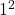## The function g(x) is defined by the equation g(x) = -3x² + x – 1. Which is the value of g(1)?

Question

The function g(x) is defined by the equation g(x) = -3x² + x – 1.
Which is the value of g(1)?

in progress 0
7 months 2021-08-10T23:45:04+00:00 1 Answers 4 views 0

-3

Step-by-step explanation:

g(1) = -3x² + x – 1

= -3() + 1 -1

= -3(1) + 1 – 1

= -3 +1 -1

g(1) = -3

Just put the 1 everywhere you see an x.

Solve